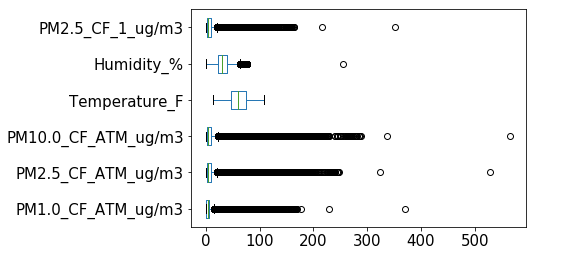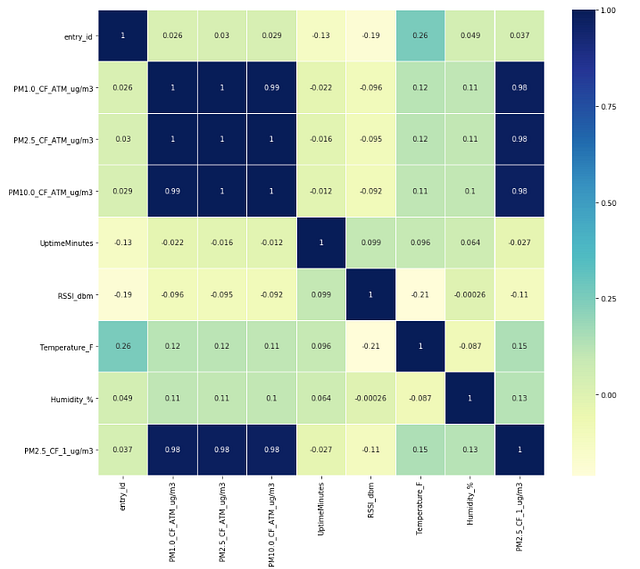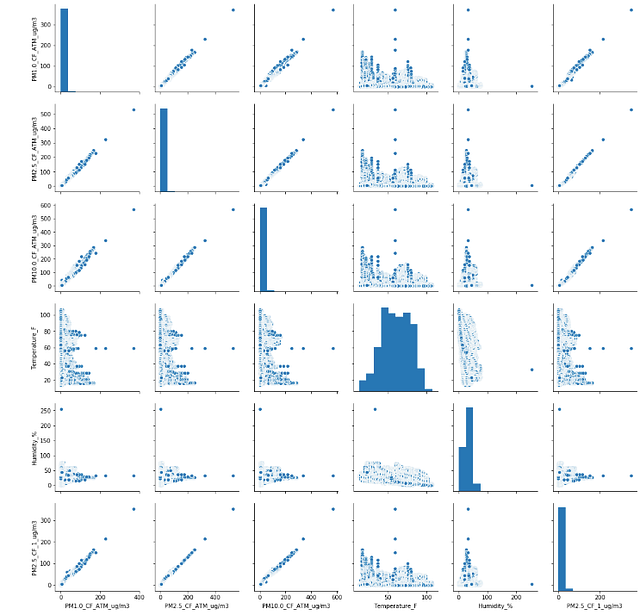#This is the third article in a series about how to take your data science projects to the next level by using a methodological approach similar to the scientific method coined the Data Science Method. This article is focused on the number of step three Exploratory Data Analysis. If you missed the previous article(s) in this series, you can go to the beginning here, or click on each step title below to read a specific step in the process.

The Data Science Method

1. Problem Identification
2. Data Collection, Organization, and Definitions
3. Exploratory Data Analysis
4. Pre-processing and Training Data Development
5. Fit Models with Training Data Set
6. Review Model Outcomes — Iterate over additional models as needed.
7. Identify the Final Model
8. Apply the Model to the Complete Data Set
9. Review the Results — Share your findings
10. Finalize Code and DocumentationCamp 3 EDA

EXPLORATORY DATA ANALYSIS (EDA)

Step number three in the Data Science Method (DSM) assumes that both steps one and two have already been completed. At this point in your data science project, you have a well-structured and defined hypothesis or problem description. The model development data set is up and ready to be explored, and your early data cleaning steps are already completed. At a minimum, you have one column per variable and have a clear understanding of your response variable.

Based on step two in the DSM you have already reviewed the following items about each variable in your data:

1. Column Name
2. The range of Values or Codes
3. Data Type (numeric, categorical, timestamp, etc.)
4. Description of Column
5. Count or percent per unique Value or Code
6. Number if NA or missing value

There are many sub-steps in a proper exploratory data analysis (EDA) workflow. Depending on your familiarity with your data and the complexity of the data and the problem you are solving the scale of the EDA necessary may change. Generally, the exploratory analysis workflow can be broken down into four critical steps:

1. Data Cleaning
2. Build data profile tables and plots
3. Explore data relationships
4. Identification and creation of features

1. DATA CLEANING

Apply NA, null, or missing value mitigation

Based on your previous work this is the point you should decide how to handle the missing, NA, or null data values, which we will refer to as “missing” from here on. If 50% or more of the rows are missing and the variable is not required to be in the model, then you should drop the variable altogether. If you have too few variables to consider dropping the one in the review, then you can use an imputation method to impute the missing values. Imputation relies on the remaining values in the variable and sometimes other variable values as well to fill the missing value with data. Before applying imputation make sure you fully understand how the imputation method you are using works so that you can identify any issues in your modeling outcome.

Drop duplicate rows and columns

Next, we need to check for duplicate rows and columns. Duplicate rows could be legitimate values depending on your data and how it was collected or the magnitude of variation that is expected in your data. The only time you should delete duplicate rows is if you are confident they are repeated measures of the same observation and that it has negative consequences for your expected statistical modeling method. Your prior work on data definitions will inform you of any duplicate columns. Duplicate columns are common when multiple data sources are combined to create the model development dataset. These may not have the same column name, but if the columns’ rows are identical to another column, one of them should be removed.

2. DATA PROFILES — PLOTS AND TABLES

Reviewing summary statistics

Summary statistics can be evaluated via a summary statistics table and by checking the individual variable distribution plots. Both will indicate the spread of your data. Depending on the distribution, you may be able to infer the mean from distribution plots; however, the summary table is the best way to review this value. Compare the example summary statistics table and the histogram plots for reference.

```#summary stats table transposed for dfdf.describe().T
```
Summary Statistics Table
`#histograms for each variable in dfhist = df.hist(bins=10,figsize =(10,10))`
Histogram plots of each variable in the data frame
Categorical variables require a slightly different approach to review the overall number of each unique value per variable and compare them to each other. The example data we are using for these figures do not contain categorical variables; however, below is an example workflow for categorical variables:
`#select categorical variables onlydf_cat = dataset.select_dtypes(include = 'object').copy()`
```#get counts of each variable value
df_cat.ColumnName.value_counts()```
```#count plot for one variable
sns.countplot(data = df_cat, x = 'ColumnName')```

Reviewing for Outliers and Anamolies

Boxplots are a quick way to identify outlier or anomalous observations. Considering these values within the context of your data is important. There may be situations where the so-called outliers or extreme values are the observations of the most interest. For example, if we review the air quality dataset used in the example summary table and histograms we see that several observations beyond the upper whisker; however, these extreme values are observations where the concentration of the particle in question probably exceeds the healthy limit. Similarly, when we review the humidity data, we have a few data points falling outside the upper limit. We want to consider if those values are data collection errors (which is very likely for anything above 100%) and then remove those observations.

`#create a boxplot for every column in dfboxplot = df.boxplot(grid=False, vert=False,fontsize=15)`Boxplot of each variable in the data frame

3. DATA RELATIONSHIPS

Investigating variable relationships through covariance matrices and other analysis methods is essential for not only evaluating the planned modeling strategy but also allows you to understand your data further. Below, we calculated the correlation coefficients for each variable in the data frame and then fed those correlations into a heatmap for ease of interpretation.Pearson Correlation Heatmap

```#create the correlation matrix heat mapplt.figure(figsize=(14,12))
sns.heatmap(df.corr(),linewidths=.1,cmap="YlGnBu", annot=True)
plt.yticks(rotation=0);```

A glance at the correlation heatmap (Figure X) shows how strongly correlated the different air pollution metrics are with each other, with values between 0.98 and 1. Logically, we know they will be highly correlated, and that is not of concern here. But, if we weren’t expecting that and were planning to treat them as independent variables in our modeling process, we would violate co-linearity rules and would need to consider using a modeling technique such as a random forest or a decision tree, which are not negatively impacted by high variable correlations.

Pair plots

Another way to evaluate the variable distributions against each other is with the seaborn pair plots function.`#pair plotsg = sns.pairplot(df)`

4. IDENTIFYING AND CREATING FEATURES

Variables and features are almost synonymous. The primary difference tends to be the context in which they are used; in machine learning, it is common practice to identify predictive features in your data whereas in parametric statistics, features are often referred to as variables and variables can include the response variable which you predict with your model.

The goal of identifying features is to use your exploratory work to isolate features that will be most helpful in constructing a predictive model. In addition to recognizing those features, it often behooves one to create additional features for inclusion in your predictive modeling work.

Once you have identified the critical features for your model development, you may realize you need to create additional features to augment your original data. You can do this through the development of combining features or revaluing them to emphasize specific relationships. Additional features can also be created through Principal Components Analysis or Clustering.

Building a Principle Components Analysis (PCA) is a useful way to apply a dimension reduction application to identify which features contain the most amount of variation within your development dataset. The predictive model can be constructed on the principal components themselves as features, resulting in feature reduction. Feature reduction is helpful when your data set has too many features to choose from, and you need a more automated way to reduce the number of input features for modeling. There are different flavors of dimension reduction methods based on multi-dimensional scaling, such as Principal Coordinate Analysis. Lasso regression is another tool for a semi-automated feature selection approach. Review these methods to determine the best strategy for your project.

Clustering (e.g. K-means clustering) is an excellent exploratory analysis method for creating additional features which in this case would be the clusters themselves. The clusters can be used in conjunction with additional features if you find them to be valid after review.

SUMMARY

Exploratory data analysis (EDA) is often an iterative process where you pose a question, review the data, and develop further questions to investigate before beginning model development work. Think of it as the process by which you develop a deeper understanding of your model development data set and prepare to develop a solid model. Often, the amount or depth of EDA is dependent on timelines for model production so expect this to vary per your data or project complexity and final modeling outcome. There is also no reason to spend hours upon hours performing EDA on a limited scope problem — use forward thinking in the processing steps taken for EDA.

In upcoming articles, I’ll cover Pre-processing and Training Data Development. Stay in touch by following me on Medium or Twitter.

Originally posted here

Views: 12295

Comment

Join Data Science CentralComment by Jessica Tanon on May 31, 2020 at 11:13pm

Hello ! Is there another page with new content for this ? This was quite interesting !